# When interaction is not interaction: confounding and measurement error

Last week, I presented ggdag at JSM in Vancouver. As you can imagine, I had a lot of conversations with people about DAGs, confounding, colliders, and all the types of bias that can arise in research. One strange type of bias came up a couple of times that I don’t see discussed very often: measuring either the effect you are studying (`x`) or a variable along a confounding pathway (`z`) incorrectly can make it appear as if there is an interaction between `x` and `z`, even if there isn’t one.

Let’s consider a simple example: there’s an association between `x` and `y`, which is what we’re interested in, and this association is confounded by `z`. This is a classic example of confounding.

``````library(broom)
library(tidyverse)
library(kableExtra) # to add headers on kable() tables
library(knitr)
library(ggdag)
library(patchwork) # to combine the plots
options(knitr.kable.NA = "--") # don't show NA values in kable()

set.seed(293951)``````
``````confounder_triangle(x_y_associated = TRUE) %>%
ggdag() +
theme_dag()``````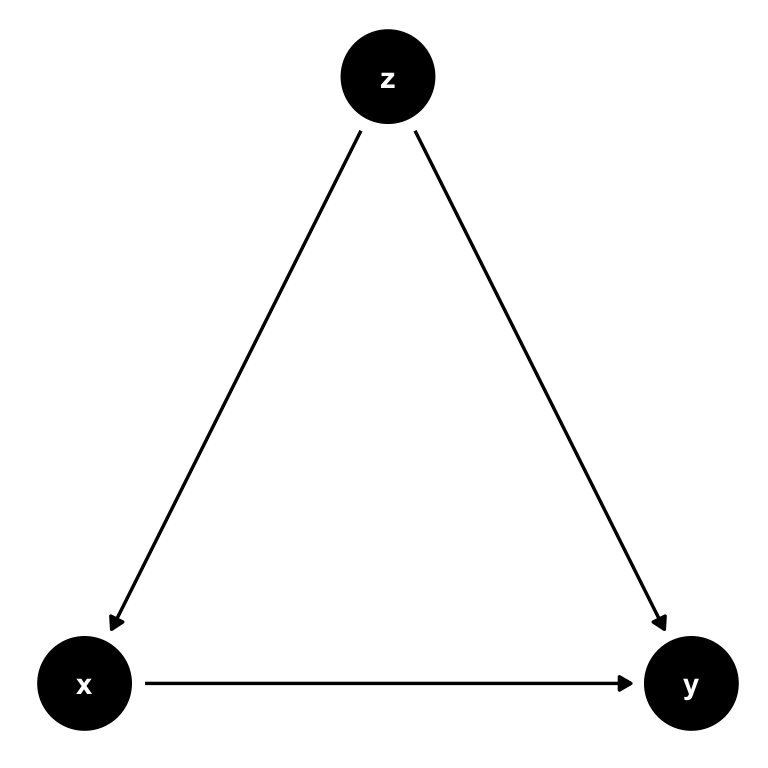Let’s simulate some data. `x` and `y` are both continuous, and `z` is binary (0 or 1, with only about 10% of the population with `z=1`). We’ll simulate 10,000 participants so random error is not a big issue.

``````z <- sample(0:1, 10000, replace = TRUE, prob = c(.9, .1))
x <- rnorm(10000) + 2*z
y <- 1 + 2*x + 1.5*z + rnorm(10000)

df <- data_frame(y, x, z)``````
``````## Warning: `data_frame()` is deprecated, use `tibble()`.
## This warning is displayed once per session.``````
``df``
``````## # A tibble: 10,000 x 3
##          y       x     z
##      <dbl>   <dbl> <int>
##  1 -0.494  -0.463      0
##  2 -0.649   0.0242     0
##  3  2.50   -0.146      0
##  4  1.36    0.0434     1
##  5  3.41    1.00       0
##  6 -2.17   -1.94       0
##  7  0.989   0.209      0
##  8  0.0667 -0.831      0
##  9  1.98    0.0196     0
## 10  1.45    0.0405     0
## # … with 9,990 more rows``````

We’ll consider what happens when we mismeasure `x` or `z`. For each scenario, we’ll compare models with and without an interaction term (`x*z`). To fit, tidy, and compare the models, let’s write a few functions:

• `tidy_lm()` tidies the regression models and formats the numbers a little
• `models_kable()` joins two tables and renders them with `kable()` using the `kableExtra` package to add a couple of headers
• `compare_models()` combines these two functions and accepts the variables we want to change as arguments so we don’t need to rewrite formulas every time
• `plot_models()` plots the relationship between `x` and `y` by levels of `z` with hex bins (for the distribution) and regression lines (to assess interaction), combining them with the `patchwork` and `cowplot` packages (plus a little tidy eval magic!)
``````tidy_lm <- function(model) {
model %>%
tidy() %>%
mutate(p.value = ifelse(p.value < .001, "<.001", round(p.value, 3))) %>%
mutate_if(is.numeric, round, 2)
}

models_kable <- function(no_int, int, ...) {
no_int <- select(no_int, term, estimate, p.value)
int <- select(int, term, estimate, p.value)
full_join(no_int, int, by = "term") %>%
mutate(term = c("(Intercept)", "x", "z", "x * z")) %>%
kable(col.names = c("Term", rep(c("Estimate", "P-Value"), 2)), ...) %>%
add_header_above(c(" ", "No Interaction" = 2, "Interaction" = 2))
}

compare_models <- function(exposure = "x", confounder = "z", ...) {
fmla <- as.formula(paste("y ~ ", exposure, " + ", confounder)) # create a formula
no_int <- lm(fmla, data = df) %>%
tidy_lm()

fmla_int <-   fmla <- as.formula(paste("y ~ ", exposure, " * ", confounder))
int <- lm(fmla_int, data = df) %>%
tidy_lm()

models_kable(no_int, int, ...)
}

plot_models <- function(x = x,
z = z,
x_label = "x (Measured well)",
z_label = "Confounder (Measured well)",
crude = FALSE) {
x <- rlang::enquo(x)
z <- rlang::enquo(z)

p1 <- df %>%
ggplot(aes(x = !!x, y = y, col = factor(!!z))) +
geom_hex(aes(fill = factor(z)), col = "white", alpha = .7) +
scale_color_manual(name = z_label, values = c("#56B4E9", "#EFA722", "#E36A25")) +
scale_fill_manual(name = z_label, values = c("#56B4E9", "#EFA722", "#E36A25")) +
theme_minimal(base_size = 14) +
theme(legend.position = "bottom",
axis.title.x = element_blank()) +
labs(y = "y (Measured well)") +
ylim(c(-10, 16))

legend <- cowplot::get_legend(p1)

p1a <- p1 + theme(legend.position = "none")

p2 <- df %>%
ggplot(aes(x = !!x, y = y, col = factor(!!z))) +
geom_hex(fill = "grey92", col = "white", alpha = .8) +
geom_smooth(method = "lm", se = FALSE, size = 1) +
scale_color_manual(name = z_label, values = c("#56B4E9", "#EFA722", "#E36A25")) +
theme_minimal(base_size = 14) +
theme(legend.position = "none",
axis.title = element_blank()) +
ylim(c(-10, 16))

if (crude) {
p2 <- p2 +
geom_smooth(aes(group = 1, col = "Crude Estimate"),
method = "lm", se = FALSE, size = 1) +
theme(legend.position = "bottom")
legend <- cowplot::get_legend(p2)
p2 <- p2 + theme(legend.position = "none")
}

patchworked <- p1a + p2
pl1 <- cowplot::plot_grid(patchworked, align = "h")
pl2 <- cowplot::add_sub(pl1, x_label,
y = 4.5, x = .5, vjust = 4.5)
pl3 <- cowplot::plot_grid(NULL, legend, NULL, nrow = 1)
cowplot::plot_grid(pl2, pl3, ncol = 1, rel_heights = c(1.5, .2))
}``````

If all three variables are measured well, there’s no problem. The effect estimates are about right, and there’s no false interaction. For the plot, notice how 1) the crude estimate (which ignores `z`) is an mix of the other two lines and 2) the slopes of the lines for `z=0` and `z=1` are parallel.

``compare_models()``
No Interaction
Interaction
Term Estimate P-Value Estimate P-Value
(Intercept) 0.99 <.001 0.99 <.001
x 2.01 <.001 2.02 <.001
z 1.46 <.001 1.47 <.001
x * z 0.00 0.912
``plot_models(crude = TRUE)``
``````## Warning: Computation failed in `stat_binhex()`:
## Package `hexbin` required for `stat_binhex`.
## Please install and try again.

## Warning: Computation failed in `stat_binhex()`:
## Package `hexbin` required for `stat_binhex`.
## Please install and try again.

## Warning: Computation failed in `stat_binhex()`:
## Package `hexbin` required for `stat_binhex`.
## Please install and try again.``````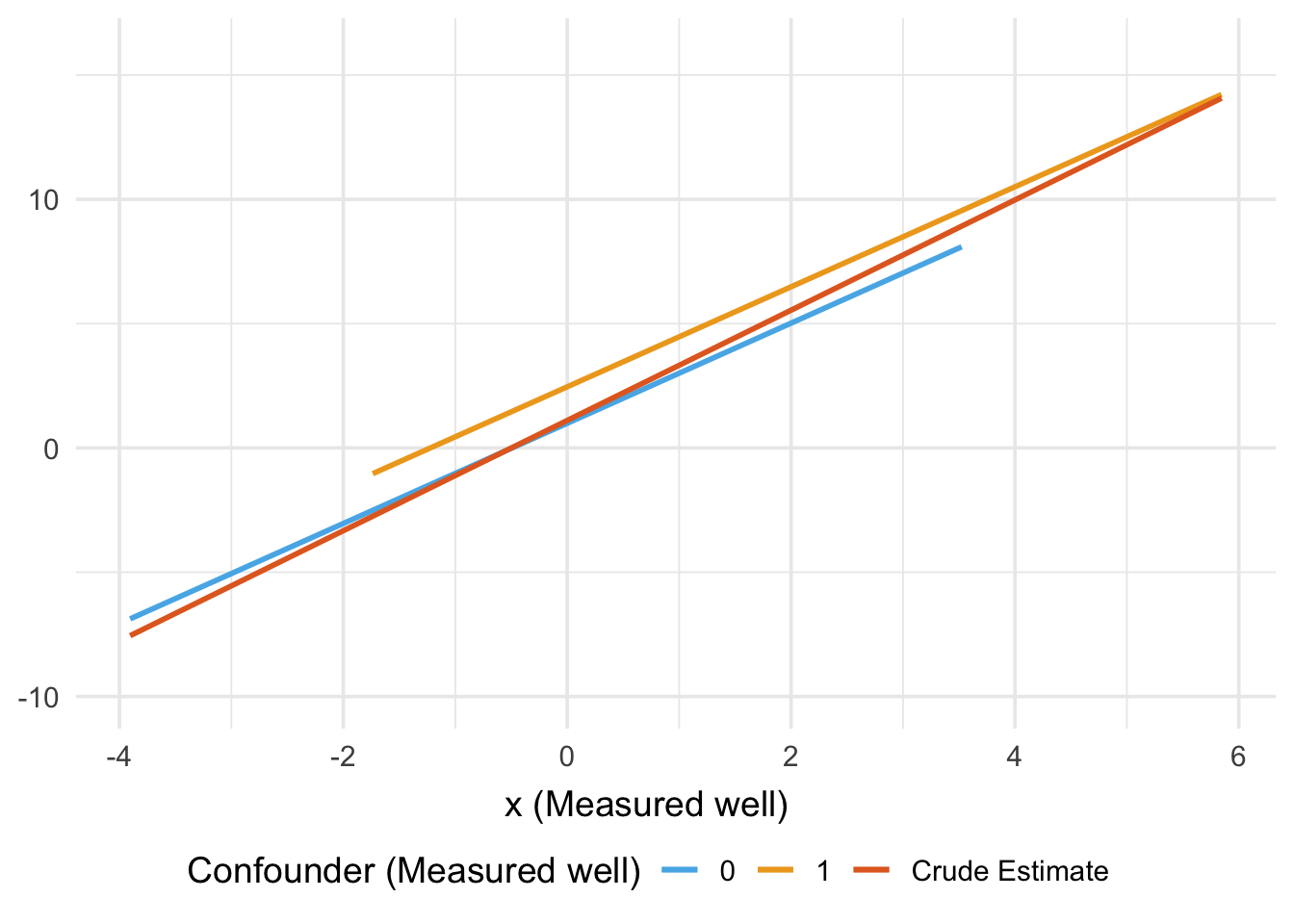## Measurement error for the outcome (`x`)

Let’s say that, in practice, we are measuring `x` incorrectly (measurement error). The only device around to measure `x` is an old 1980 version of the measureator, which by complete coincidence is the same year Sander Greenland first talked about this issue. The 1980 version of this device has a problem: it measures `x` better in people who have values of `y` above 0 than those below 0. The 2018 version of the device still measures `x` with error, but the manufacturer fixed the problem with `y`, and now it has nothing to do with that.

### Mismeasured `x`, dependent on `y`

When we use `x_1980` as a proxy for `x`, there now appears to be an interaction with `z`. It’s present in both the model estimates and the plot. The slopes are no longer parallel. The effect of `x` on `y` seems like it’s heterogeneous for levels of `z`: for people with `z=1`, the effect of `x` on `y` is stronger.

``````measureator2018 <- function(x) x + rnorm(10000)
measureator1980 <- function(x) ifelse(y > 2, x + rnorm(10000, sd = 1.5), ifelse(y > 0, x + rnorm(10000, sd = 2), x + rnorm(10000, sd = 3)))

df <- df %>%
mutate(
x_2018 = measureator2018(x),
x_1980 = measureator1980(x)
)

compare_models("x_1980")``````
No Interaction
Interaction
Term Estimate P-Value Estimate P-Value
(Intercept) 0.98 <.001 0.98 <.001
x 0.34 <.001 0.32 <.001
z 4.80 <.001 4.28 <.001
x * z 0.29 <.001
``plot_models(x = x_1980, x_label = "Mismeasured x (1980 device)")``
``````## Warning: Computation failed in `stat_binhex()`:
## Package `hexbin` required for `stat_binhex`.
## Please install and try again.

## Warning: Computation failed in `stat_binhex()`:
## Package `hexbin` required for `stat_binhex`.
## Please install and try again.``````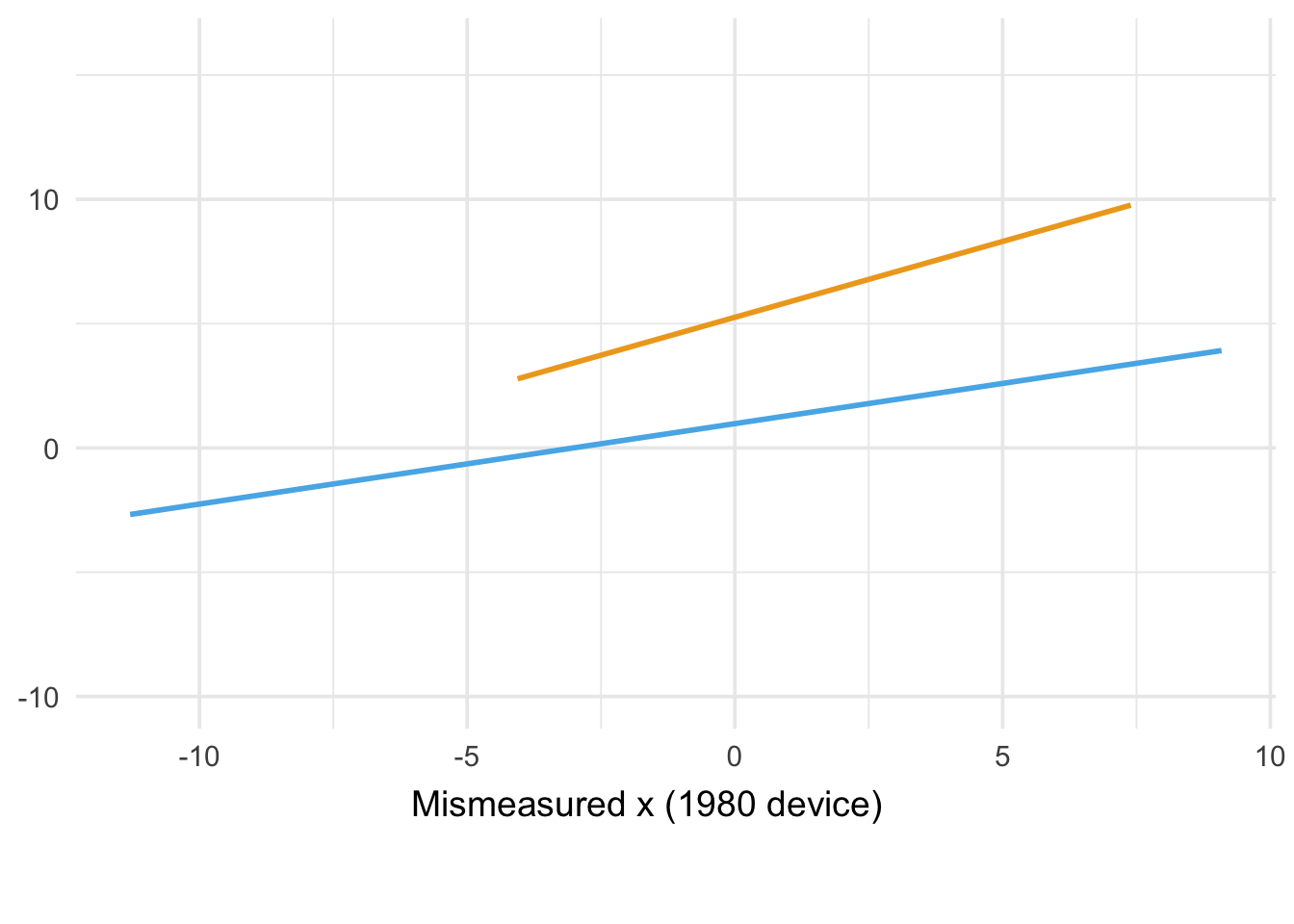What’s going on here? The plot helps demonstrate the distortion in x (it’s much less stable when `y` is below 0 and most people with `z=1` are above 0). We can put this together in a DAG. In the case of the 1980 device, we have differential measurement error, so called because the degree of mismeasurement is affected by `y`. We want to estimate the effect of `x`, but what we really have is an approximation, `x_m`, dependent on both `error` (the amount of mismeasurement) and `x`. `error` is dependent on `y`.

``````dme <- dagify(y ~ x + z,
x ~ z,
x_m ~ x + error,
error ~ y)

ggdag(dme) +
theme_dag()``````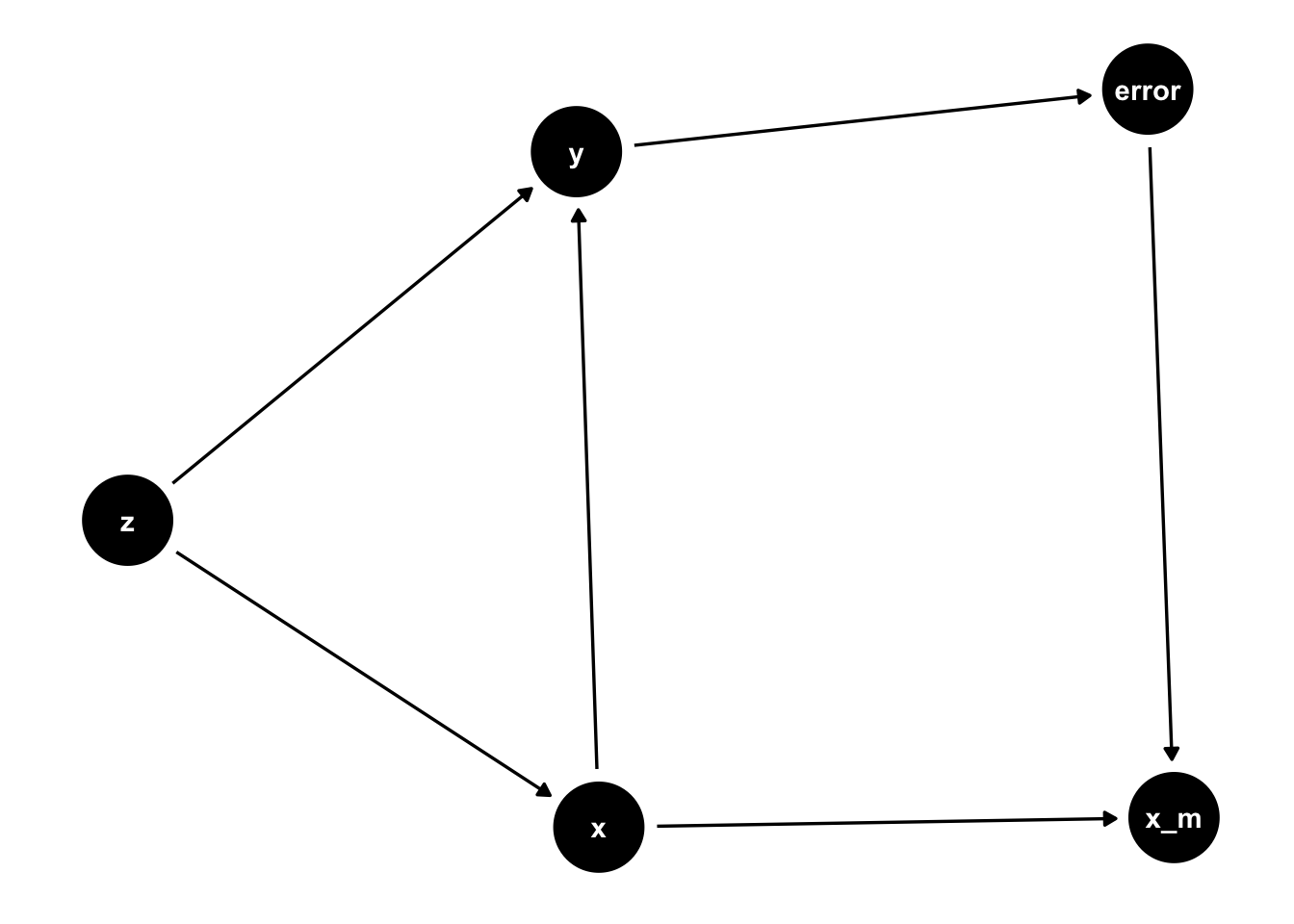Because two arrows are leading into it, `x_m` is a collider. Including a collider in the regression model will induce an association between its parents, creating bias. Collider bias can also travel upstream (see the previous link), here affecting `z` and `y`, as well. Look at the web of associations it creates!

``````ggdag_adjust(dme, c("x_m", "z")) +
theme_dag() +
scale_color_manual(values = c("#2C7FBF", "#E69F00")) +
ggraph::scale_edge_color_manual(values = c("#E7E7E7", "#E69F00"))``````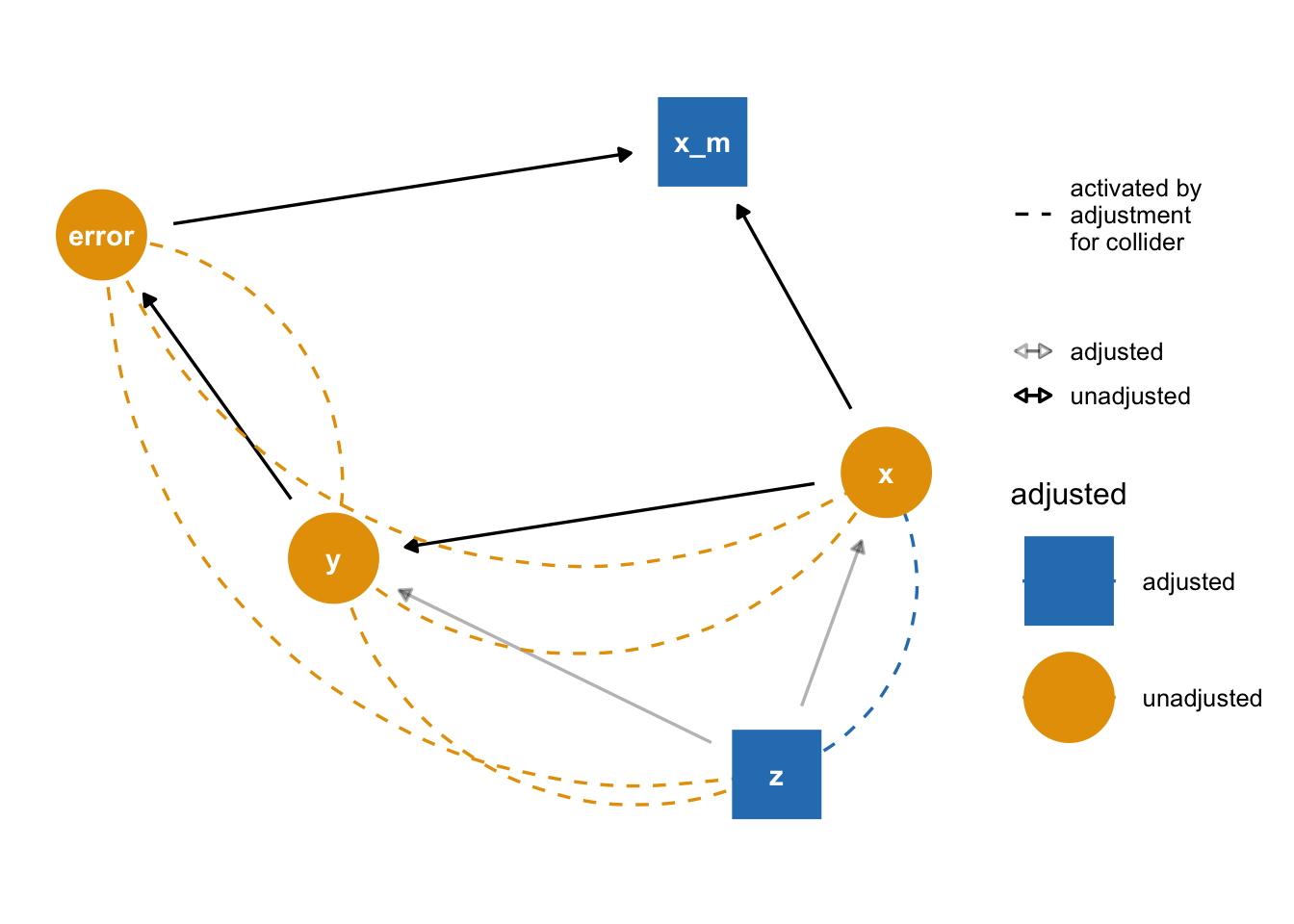### Mismeasured `x`, independent of `y`

Things are a little different if we use the 2018 device, which measures `x` incorrectly but has nothing to do with `y`. In this case, there’s no apparent interaction.

``compare_models("x_2018")``
No Interaction
Interaction
Term Estimate P-Value Estimate P-Value
(Intercept) 0.97 <.001 0.97 <.001
x 0.99 <.001 0.99 <.001
z 3.48 <.001 3.40 <.001
x * z 0.04 0.27
``plot_models(x = x_2018, x_label = "Mismeasured x (1980 device)")``
``````## Warning: Computation failed in `stat_binhex()`:
## Package `hexbin` required for `stat_binhex`.
## Please install and try again.

## Warning: Computation failed in `stat_binhex()`:
## Package `hexbin` required for `stat_binhex`.
## Please install and try again.``````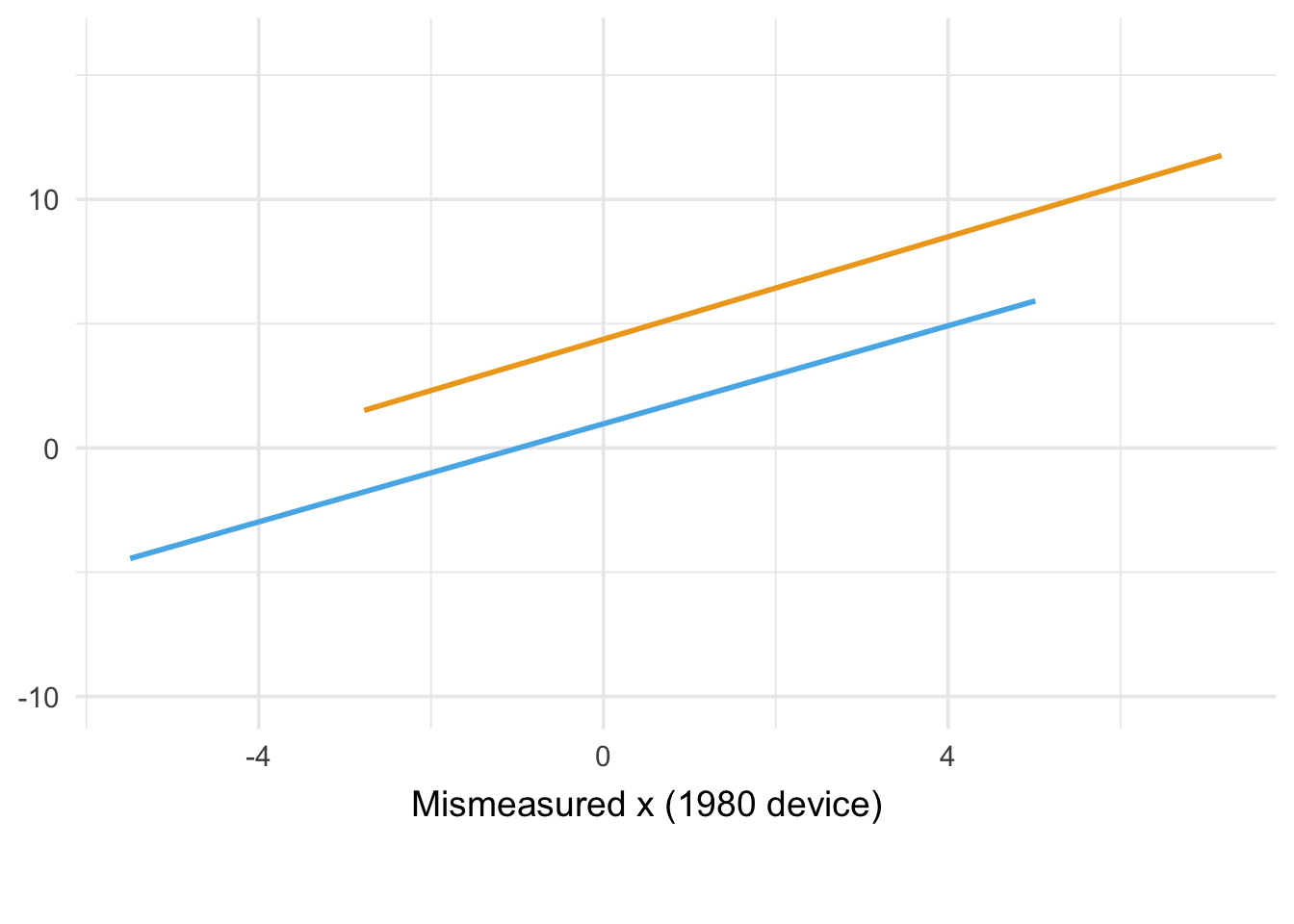This is a case of non-differential measurement error; the error is not dependent on the outcome, `y`. `x_m` is still a collider but it ends up being less important. Instead, the model underestimate the effect of `x` on `y` (otherwise known as bias towards the null, which is what happens under most cases of non-differential error) and attributes too much of the effect on `y` to `z` (`x` is a mediator of `z`’s impact on `y`).

``````ndme <- dagify(y ~ x + z,
x ~ z,
x_m ~ x + error)

ggdag(ndme) +
theme_dag()``````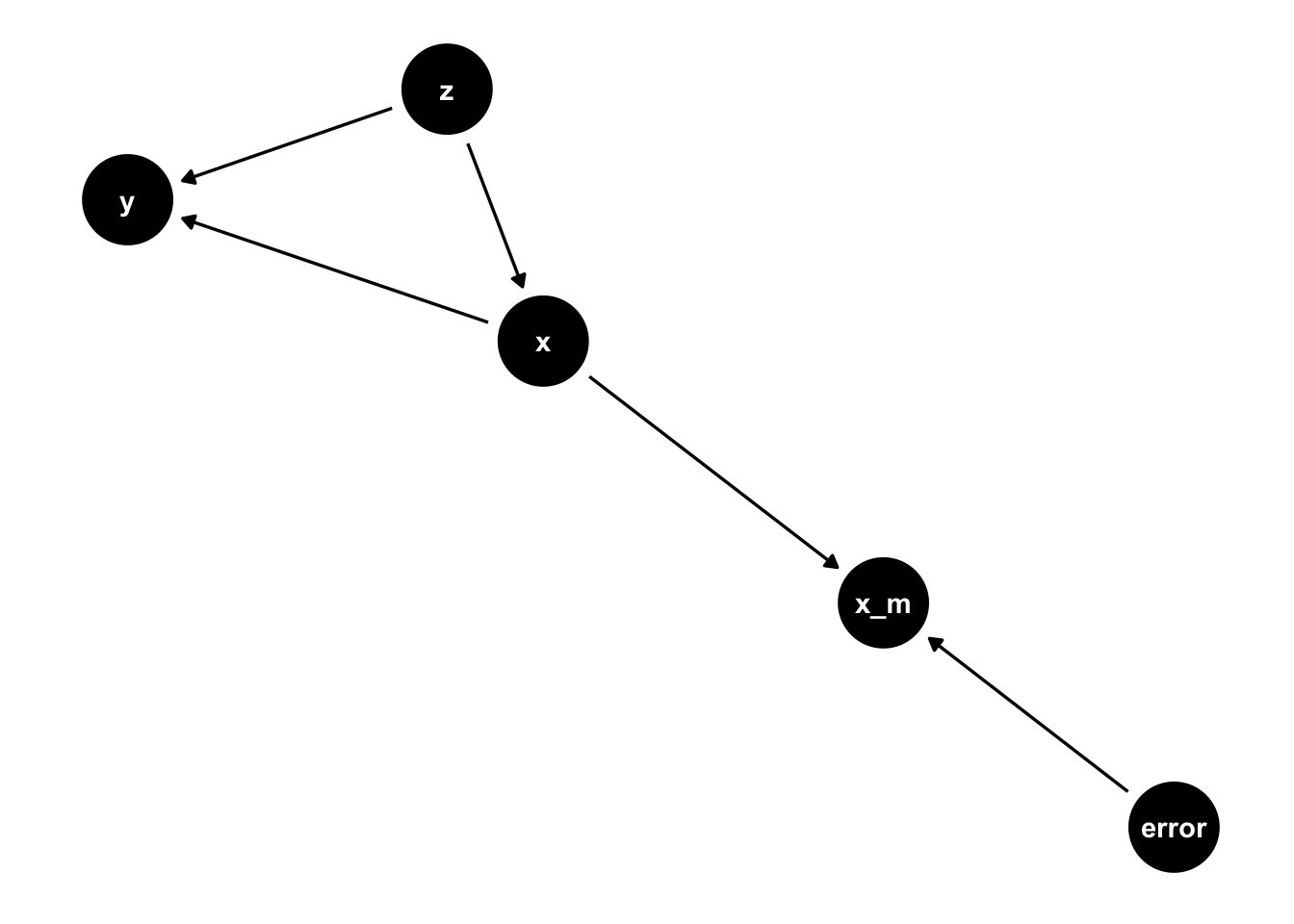## Measurement error for covariates (`z`)

What about if we measure `x` well but mismeasure the confounder, `z`? Let’s say we have a similar situation: one device that occasionally misclassifies `z` but isn’t effected by `y` and another, broken device that measures `z` in people who have higher values of `y` better.

``````swap <- function(x) ifelse(x == 1, 0, 1)

z_ometer <- function(z) {
mismeasured <- sample(c(TRUE, FALSE), size = 10000, replace = TRUE, prob = c(.3, .7))
ifelse(mismeasured, swap(z), z)
}

z_ometer_broken <- function(z) {
mismeasured <- ifelse(
df\$y > 0,
sample(c(TRUE, FALSE), size = 10000, replace = TRUE, prob = c(.5, .5)),
sample(c(TRUE, FALSE), size = 10000, replace = TRUE, prob = c(.1, .9))
)
ifelse(mismeasured, swap(z), z)
}

df <- df %>%
mutate(
z_results = z_ometer(z),
z_results_broken = z_ometer_broken(z)
)``````

The DAGs for `z_m` look pretty similar to `x_m`.

``````ndme_z <- dagify(y ~ x + z,
x ~ z,
z_m ~ z + error)

dme_z <- dagify(y ~ x + z,
x ~ z,
z_m ~ z + error,
error ~ y)

dag1 <- ggdag(ndme_z) +
theme_dag() +
ggtitle("Wrongly measure z, independent of y")

dag2 <- ggdag(dme_z) +
theme_dag() +
ggtitle("Wrongly measure z, dependent on y")

dag1 + dag2``````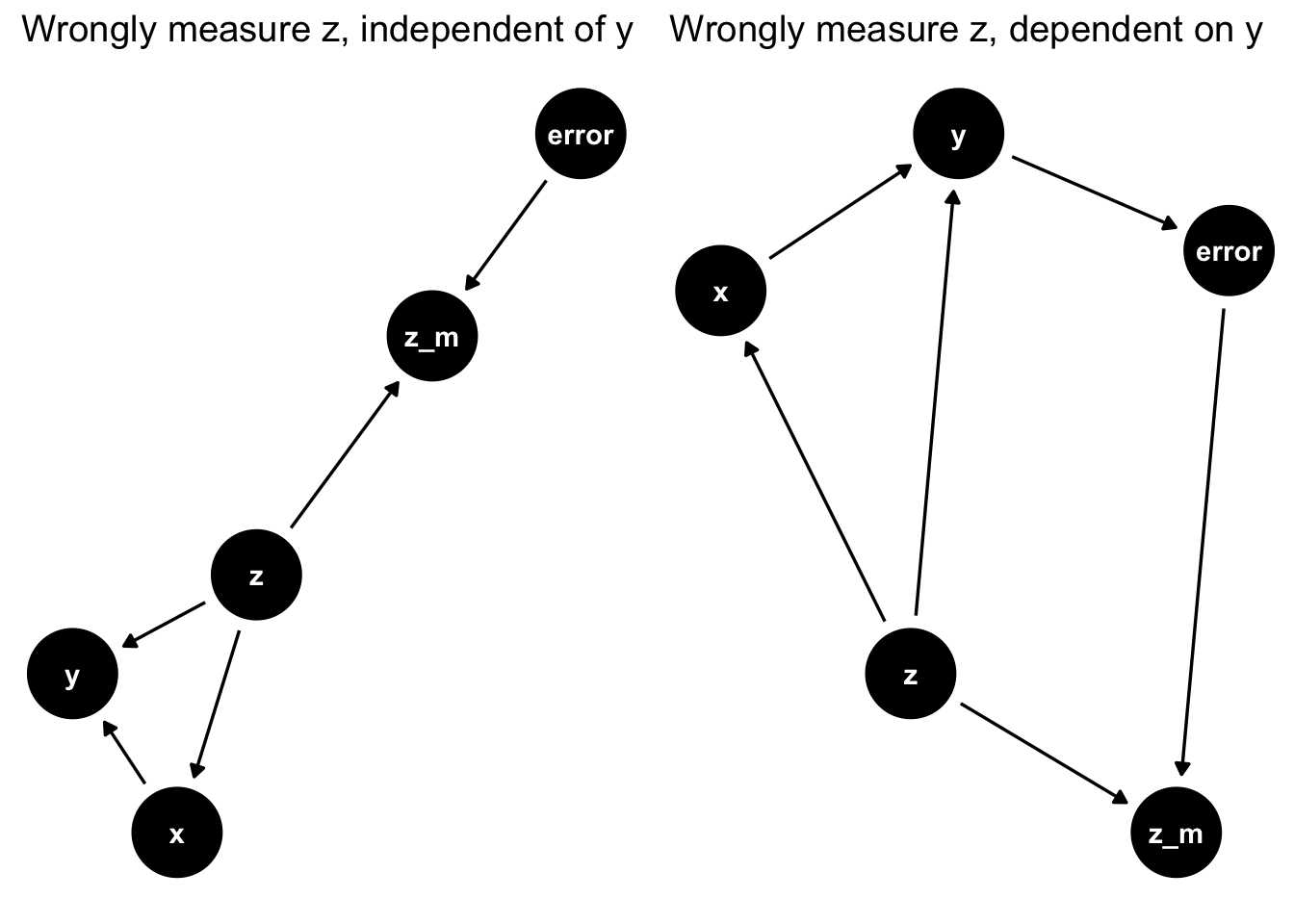For both devices, there appears to be a little interaction (probably only detectable because of our large sample size). While that’s often the case, the larger issue is that our proxies for `z` are no longer blocking the back-door path between `x` and `y`. In other words, there’s residual confounding. In this example, what ends up happening is a little bit of false interaction for both differential and non-differential misclassification of `z` and a lot of confounding bias. The estimate for `x` is a little bit off (about 10% too high), and `z` is much too low (it’s biased towards the null).

### Mismeasured `z`, dependent on `y`

``compare_models(confounder = "z_results_broken")``
No Interaction
Interaction
Term Estimate P-Value Estimate P-Value
(Intercept) 1.02 <.001 1.02 <.001
x 2.19 <.001 2.22 <.001
z 0.23 <.001 0.26 <.001
x * z -0.08 <.001
``plot_models(z = z_results_broken, z_label = "Confounder (Z-ometer, broken)")``
``````## Warning: Computation failed in `stat_binhex()`:
## Package `hexbin` required for `stat_binhex`.
## Please install and try again.

## Warning: Computation failed in `stat_binhex()`:
## Package `hexbin` required for `stat_binhex`.
## Please install and try again.``````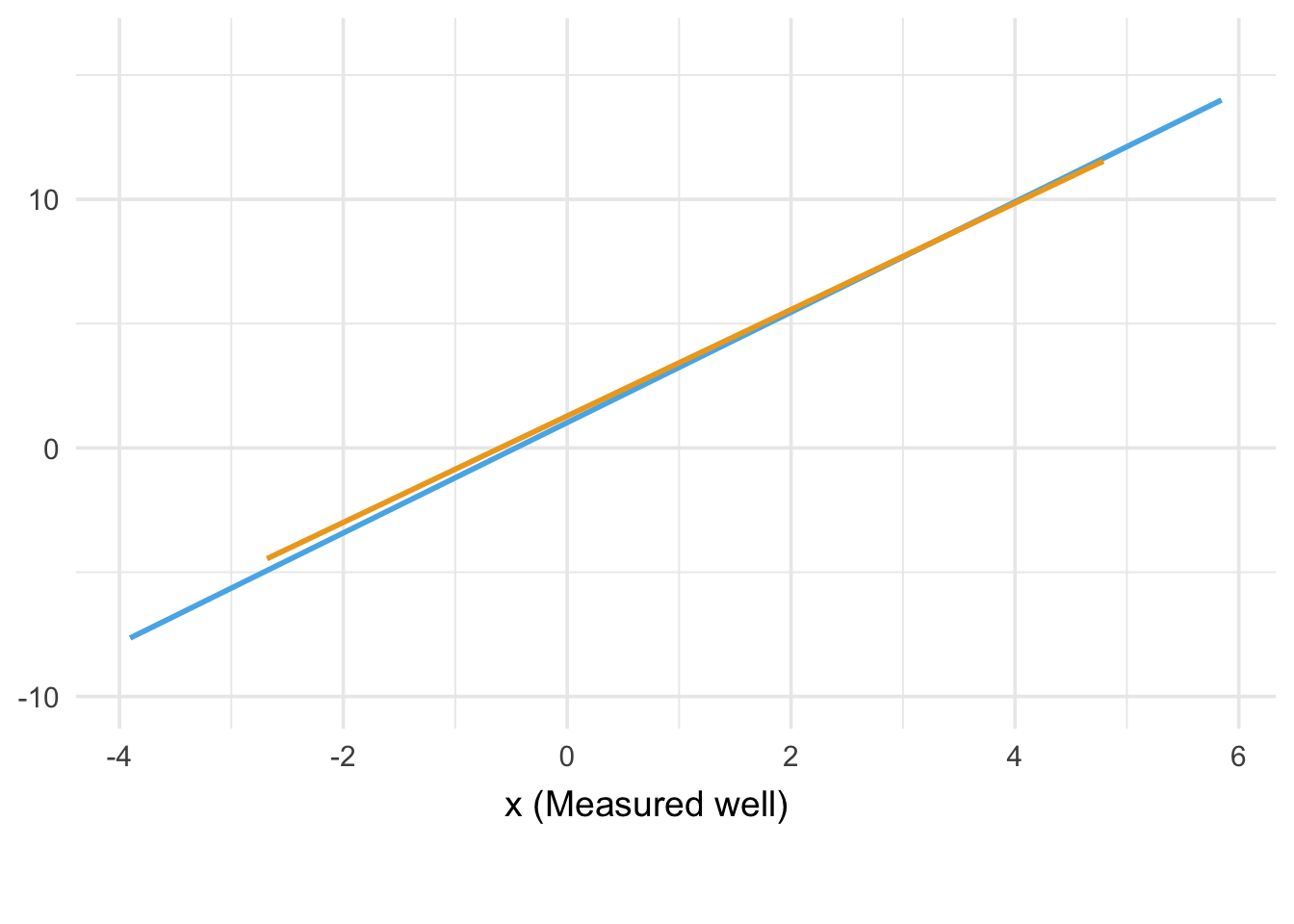### Mismeasured `z`, independent of `y`

``compare_models(confounder = "z_results")``
No Interaction
Interaction
Term Estimate P-Value Estimate P-Value
(Intercept) 1.03 <.001 1.04 <.001
x 2.21 <.001 2.13 <.001
z 0.21 <.001 0.16 <.001
x * z 0.19 <.001
``plot_models(z = z_results, z_label = "Confounder (Z-ometer, broken)")``
``````## Warning: Computation failed in `stat_binhex()`:
## Package `hexbin` required for `stat_binhex`.
## Please install and try again.

## Warning: Computation failed in `stat_binhex()`:
## Package `hexbin` required for `stat_binhex`.
## Please install and try again.``````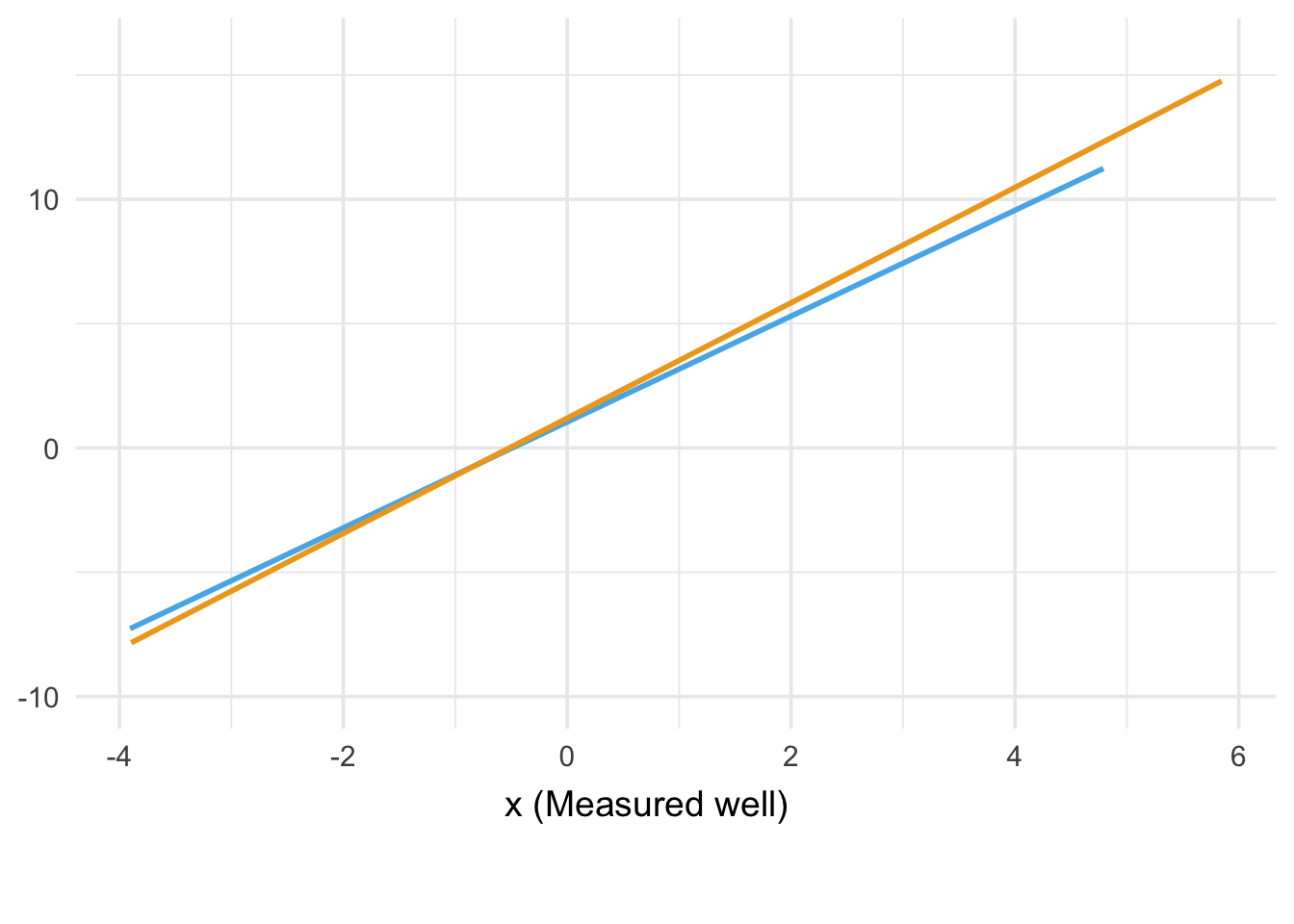So, mismeasurement of both `x` and `z` can cause problems. Under most circumstances, of course, we are mismeasuring more than one variable (including `y`!). Moreover, the errors in the way those variables are measured may themselves be dependent. We need to, then, be very mindful of the structures of these bias and, if necessary, try to address them with bias analysis approaches.

If you want to learn about more about these methods, you may be interested in this great-looking resource from Maarten van Smeden:

{{< tweet 1025990407757422592 >}}

Thanks to him for providing it!##### Malcolm Barrett
###### Data Scientist

I am a data scientist, an R developer, and an epidemiologist. My work in public health has spanned on-ground clinical education and research for clinical and cohort studies. Previously, I was an intern at RStudio, and I served two years in AmeriCorps at federally-qualified health centers in Michigan and New York City.